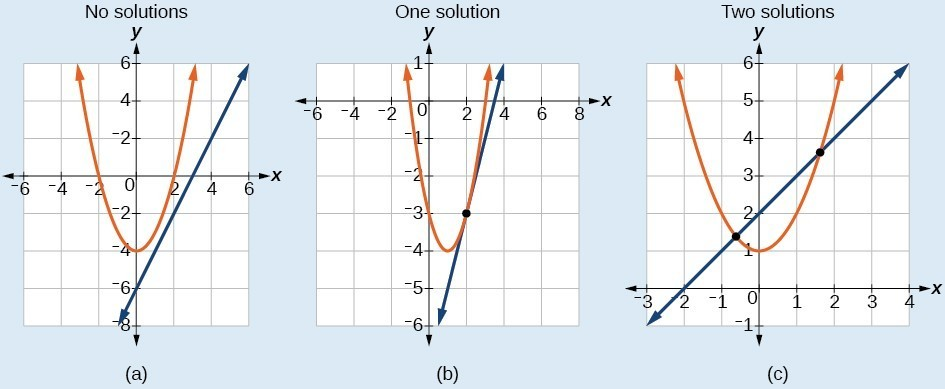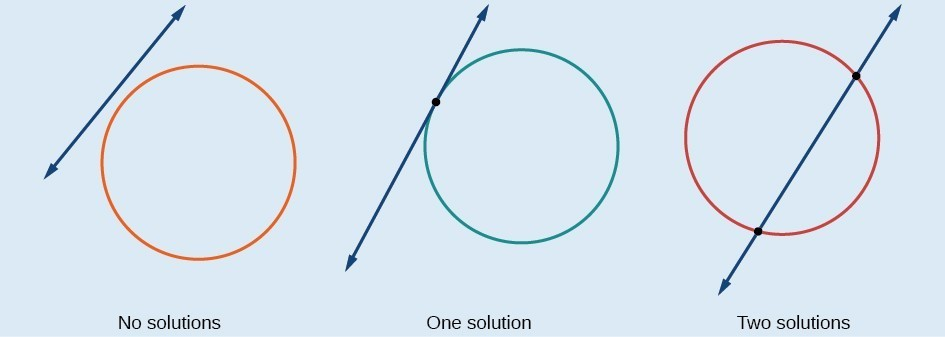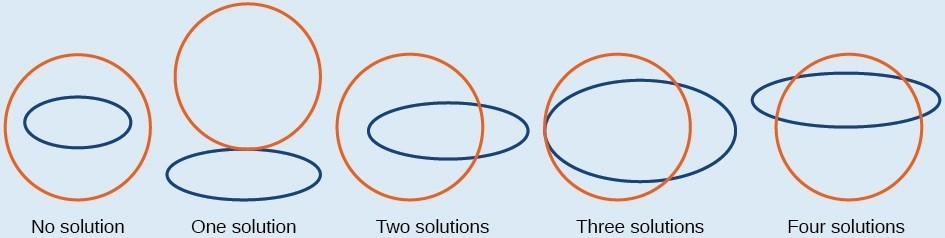## Methods for Solving a System of Nonlinear Equations

### Learning Outcomes

• Solve a system that represents the intersection of a parabola and a line using substitution.
• Solve a system that represents the intersection of a circle and a line using substitution.
• Solve a system that represents the intersection of a circle and an ellipse using elimination.

A system of nonlinear equations is a system of two or more equations in two or more variables containing at least one equation that is not linear. Recall that a linear equation can take the form $Ax+By+C=0$. Any equation that cannot be written in this form in nonlinear. The substitution method we used for linear systems is the same method we will use for nonlinear systems. We solve one equation for one variable and then substitute the result into the second equation to solve for another variable, and so on. There is, however, a variation in the possible outcomes.

## Intersection of a Parabola and a Line

There are three possible types of solutions for a system of nonlinear equations involving a parabola and a line.

### A General Note: Possible Types of Solutions for Points of Intersection of a Parabola and a Line

The graphs below illustrate possible solution sets for a system of equations involving a parabola and a line.

• No solution. The line will never intersect the parabola.
• One solution. The line is tangent to the parabola and intersects the parabola at exactly one point.
• Two solutions. The line crosses on the inside of the parabola and intersects the parabola at two points.### How To: Given a system of equations containing a line and a parabola, find the solution.

1. Solve the linear equation for one of the variables.
2. Substitute the expression obtained in step one into the parabola equation.
3. Solve for the remaining variable.
4. Check your solutions in both equations.

### Example: Solving a System of Nonlinear Equations Representing a Parabola and a Line

Solve the system of equations.

$\begin{array}{l}x-y=-1\hfill \\ y={x}^{2}+1\hfill \end{array}$

### Could we have substituted values for $y$ into the second equation to solve for $x$ in Example 1?

Yes, but because $x$ is squared in the second equation this could give us extraneous solutions for $x$.

For $y=1$

\begin{align}&y={x}^{2}+1 \\ &y={x}^{2}+1 \\ &{x}^{2}=0 \\ &x=\pm \sqrt{0}=0 \end{align}

This gives us the same value as in the solution.

For $y=2$

\begin{align}&y={x}^{2}+1 \\ &2={x}^{2}+1 \\ &{x}^{2}=1 \\ &x=\pm \sqrt{1}=\pm 1 \end{align}

Notice that $-1$ is an extraneous solution.

### Try It

Solve the given system of equations by substitution.

$\begin{gathered}3x-y=-2 \\ 2{x}^{2}-y=0 \end{gathered}$

## Intersection of a Circle and a Line

Just as with a parabola and a line, there are three possible outcomes when solving a system of equations representing a circle and a line.

### A General Note: Possible Types of Solutions for the Points of Intersection of a Circle and a Line

The graph below illustrates possible solution sets for a system of equations involving a circle and a line.

• No solution. The line does not intersect the circle.
• One solution. The line is tangent to the circle and intersects the circle at exactly one point.
• Two solutions. The line crosses the circle and intersects it at two points.### How To: Given a system of equations containing a line and a circle, find the solution.

1. Solve the linear equation for one of the variables.
2. Substitute the expression obtained in step one into the equation for the circle.
3. Solve for the remaining variable.
4. Check your solutions in both equations.

### Example: Finding the Intersection of a Circle and a Line by Substitution

Find the intersection of the given circle and the given line by substitution.

$\begin{gathered}{x}^{2}+{y}^{2}=5 \\ y=3x - 5 \end{gathered}$

### Try It

Solve the system of nonlinear equations.

$\begin{array}{l}{x}^{2}+{y}^{2}=10\hfill \\ x - 3y=-10\hfill \end{array}$

## Solving a System of Nonlinear Equations Using Elimination

We have seen that substitution is often the preferred method when a system of equations includes a linear equation and a nonlinear equation. However, when both equations in the system have like variables of the second degree, solving them using elimination by addition is often easier than substitution. Generally, elimination is a far simpler method when the system involves only two equations in two variables (a two-by-two system), rather than a three-by-three system, as there are fewer steps. As an example, we will investigate the possible types of solutions when solving a system of equations representing a circle and an ellipse.

### A General Note: Possible Types of Solutions for the Points of Intersection of a Circle and an Ellipse

The figure below illustrates possible solution sets for a system of equations involving a circle and an ellipse.

• No solution. The circle and ellipse do not intersect. One shape is inside the other or the circle and the ellipse are a distance away from the other.
• One solution. The circle and ellipse are tangent to each other, and intersect at exactly one point.
• Two solutions. The circle and the ellipse intersect at two points.
• Three solutions. The circle and the ellipse intersect at three points.
• Four solutions. The circle and the ellipse intersect at four points.### Example: Solving a System of Nonlinear Equations Representing a Circle and an Ellipse

Solve the system of nonlinear equations.

\begin{align} {x}^{2}+{y}^{2}=26 \hspace{5mm} \left(1\right)\\ 3{x}^{2}+25{y}^{2}=100 \hspace{5mm} \left(2\right)\end{align}

### Try It

Find the solution set for the given system of nonlinear equations.

$\begin{gathered}4{x}^{2}+{y}^{2}=13\\ {x}^{2}+{y}^{2}=10\end{gathered}$

In the following video, we present an example of how to solve a system of non-linear equations that represent the intersection of an ellipse and a hyperbola.